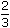# Aptitude - Time and Distance

Exercise : Time and Distance - General Questions
1.
A person crosses a 600 m long street in 5 minutes. What is his speed in km per hour?
3.6
7.2
8.4
10
Explanation:

 Speed =600m/sec. 5 x 60

= 2 m/sec.

Converting m/sec to km/hr (see important formulas section)

 =2 x 18km/hr 5

= 7.2 km/hr.

2.
An aeroplane covers a certain distance at a speed of 240 kmph in 5 hours. To cover the same distance in 1hours, it must travel at a speed of:
300 kmph
360 kmph
600 kmph
720 kmph
Explanation:

Distance = (240 x 5) = 1200 km.

Speed = Distance/Time

Speed = 1200/(5/3) km/hr.     [We can write 1hours as 5/3 hours]Required speed =1200 x 3km/hr = 720 km/hr. 5

3.
If a person walks at 14 km/hr instead of 10 km/hr, he would have walked 20 km more. The actual distance travelled by him is:
50 km
56 km
70 km
80 km
Explanation:

Let the actual distance travelled be x km.

 Then, x = x + 20 10 1414x = 10x + 2004x = 200x = 50 km.

4.
A train can travel 50% faster than a car. Both start from point A at the same time and reach point B 75 kms away from A at the same time. On the way, however, the train lost about 12.5 minutes while stopping at the stations. The speed of the car is:
100 kmph
110 kmph
120 kmph
130 kmph
Explanation:

Let speed of the car be x kmph.

 Then, speed of the train = 150 x =3 xkmph. 100 275 - 75 = 125 x (3/2)x 10 x 6075 - 50 = 5 x x 24x =25 x24= 120 kmph. 5

5.
Excluding stoppages, the speed of a bus is 54 kmph and including stoppages, it is 45 kmph. For how many minutes does the bus stop per hour?
9
10
12
20
 Time taken to cover 9 km =9 x 60min = 10 min. 54